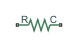# Translational Spring

Ideal spring in mechanical translational systems

## Library

Mechanical Translational Elements

•## Description

The Translational Spring block represents an ideal mechanical linear spring, described with the following equations:

`$F=Kx$`
`$x={x}_{init}+{x}_{R}-{x}_{C}$`
`$v=\frac{dx}{dt}$`

where

 `F` Force transmitted through the spring `K` Spring rate `x` Relative displacement (spring deformation) `xinit` Spring initial displacement (initial deformation); the spring can be initially compressed (```xinit > 0```) or stretched (```xinit < 0```) `xR,xC` Absolute displacements of terminals R and C, respectively `v` Relative velocity `t` Time

The block positive direction is from port R to port C. This means that the force is positive if it acts in the direction from R to C.

### Variables

To set the priority and initial target values for the block variables prior to simulation, use the Initial Targets section in the block dialog box or Property Inspector. For more information, see Set Priority and Initial Target for Block Variables.

Nominal values provide a way to specify the expected magnitude of a variable in a model. Using system scaling based on nominal values increases the simulation robustness. Nominal values can come from different sources, one of which is the Nominal Values section in the block dialog box or Property Inspector. For more information, see Modify Nominal Values for a Block Variable.

## Parameters

Spring rate

Spring rate. The default value is `1000` N/m.

## Ports

The block has the following ports:

`R`

Mechanical translational conserving port.

`C`

Mechanical translational conserving port.

## Version History

Introduced in R2007a